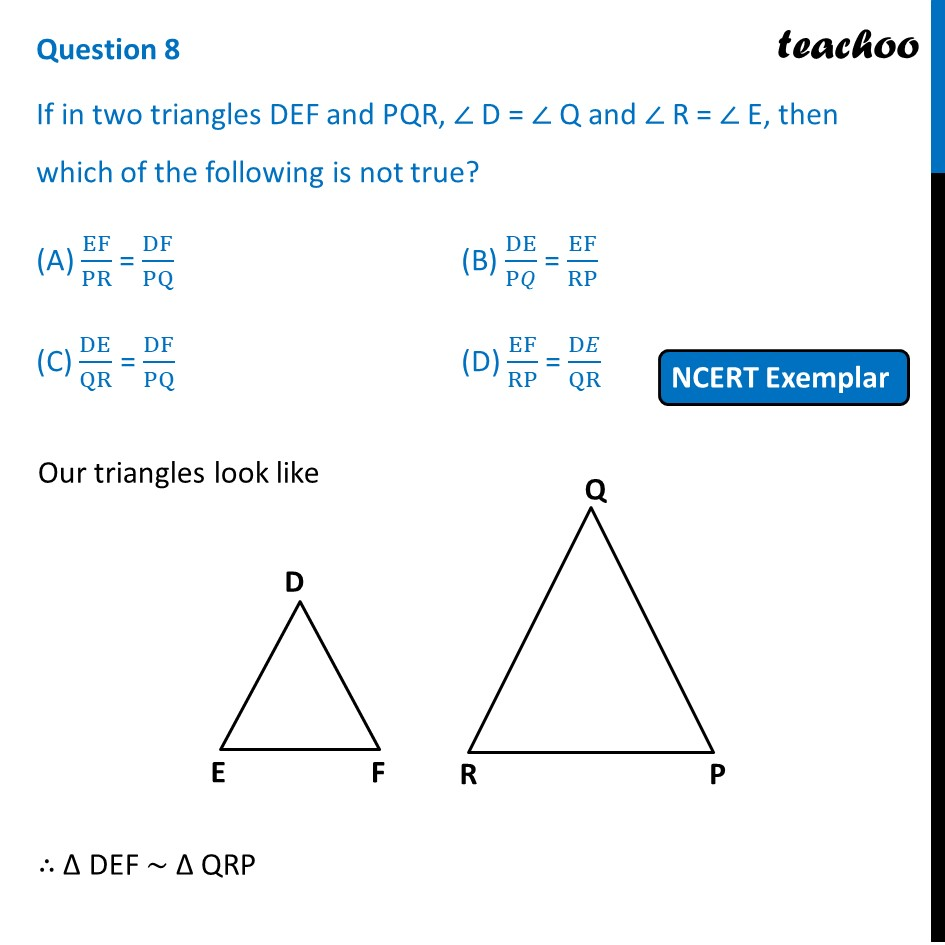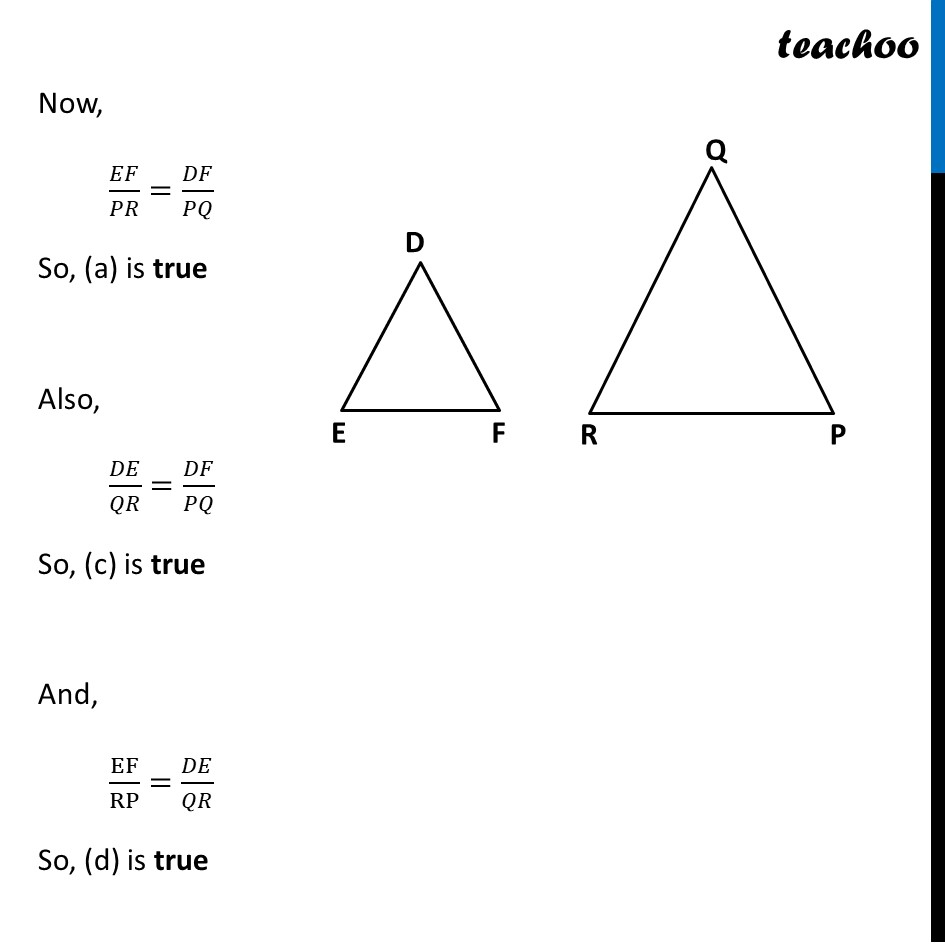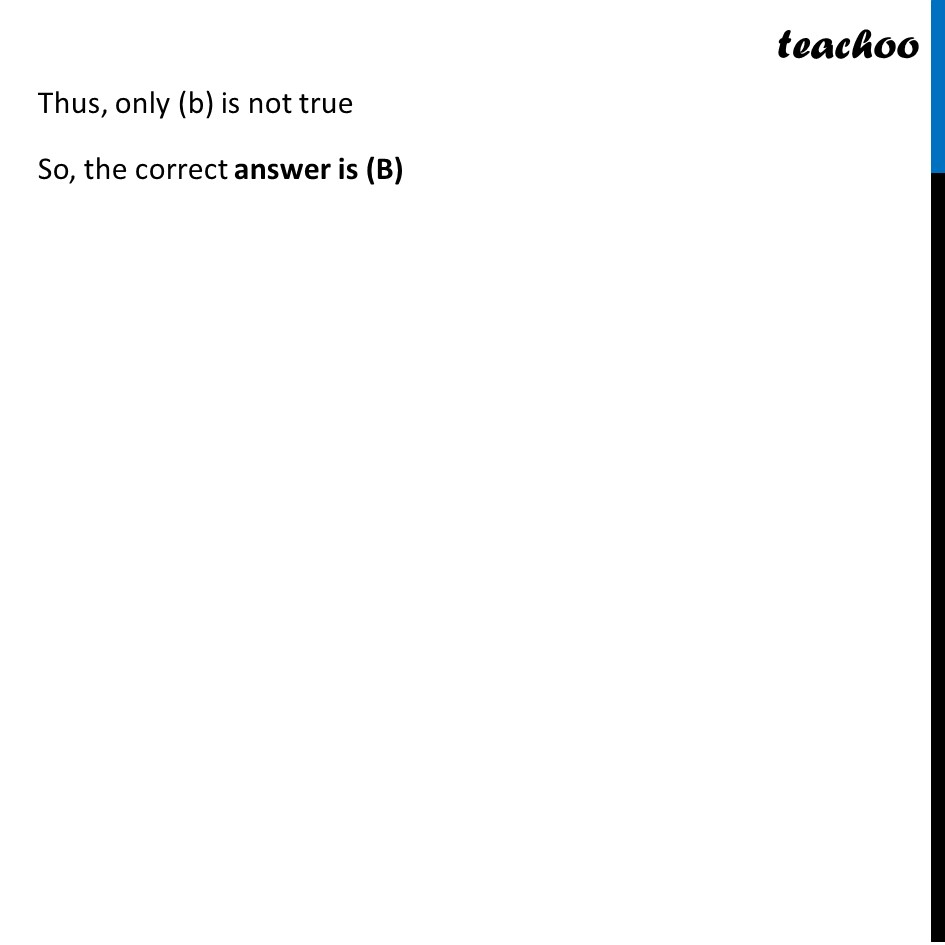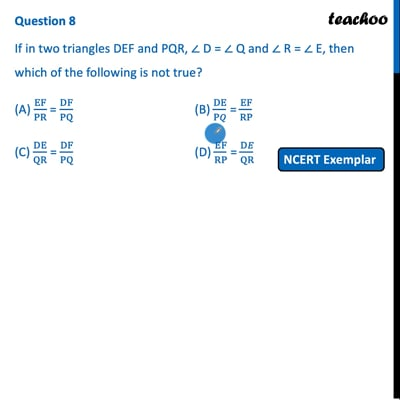NCERT Exemplar - MCQ

Chapter 6 Class 10 Triangles
Serial order wise

## (D) EF/RP = DE/QRThis video is only available for Teachoo black users

Maths Crash Course - Live lectures + all videos + Real time Doubt solving!

### Transcript

Question 8 If in two triangles DEF and PQR, ∠ D = ∠ Q and ∠ R = ∠ E, then which of the following is not true? (A) EF/PR = DF/PQ (B) DE/P𝑄 = EF/RP (C) DE/QR = DF/PQ (D) EF/RP = D𝐸/QR Our triangles look like ∴ Δ DEF ~ Δ QRP Now, 𝐸𝐹/𝑃𝑅=𝐷𝐹/𝑃𝑄 So, (a) is true Also, 𝐷𝐸/𝑄𝑅=𝐷𝐹/𝑃𝑄 So, (c) is true And, EF/RP=𝐷𝐸/𝑄𝑅 So, (d) is true Thus, only (b) is not true So, the correct answer is (B)